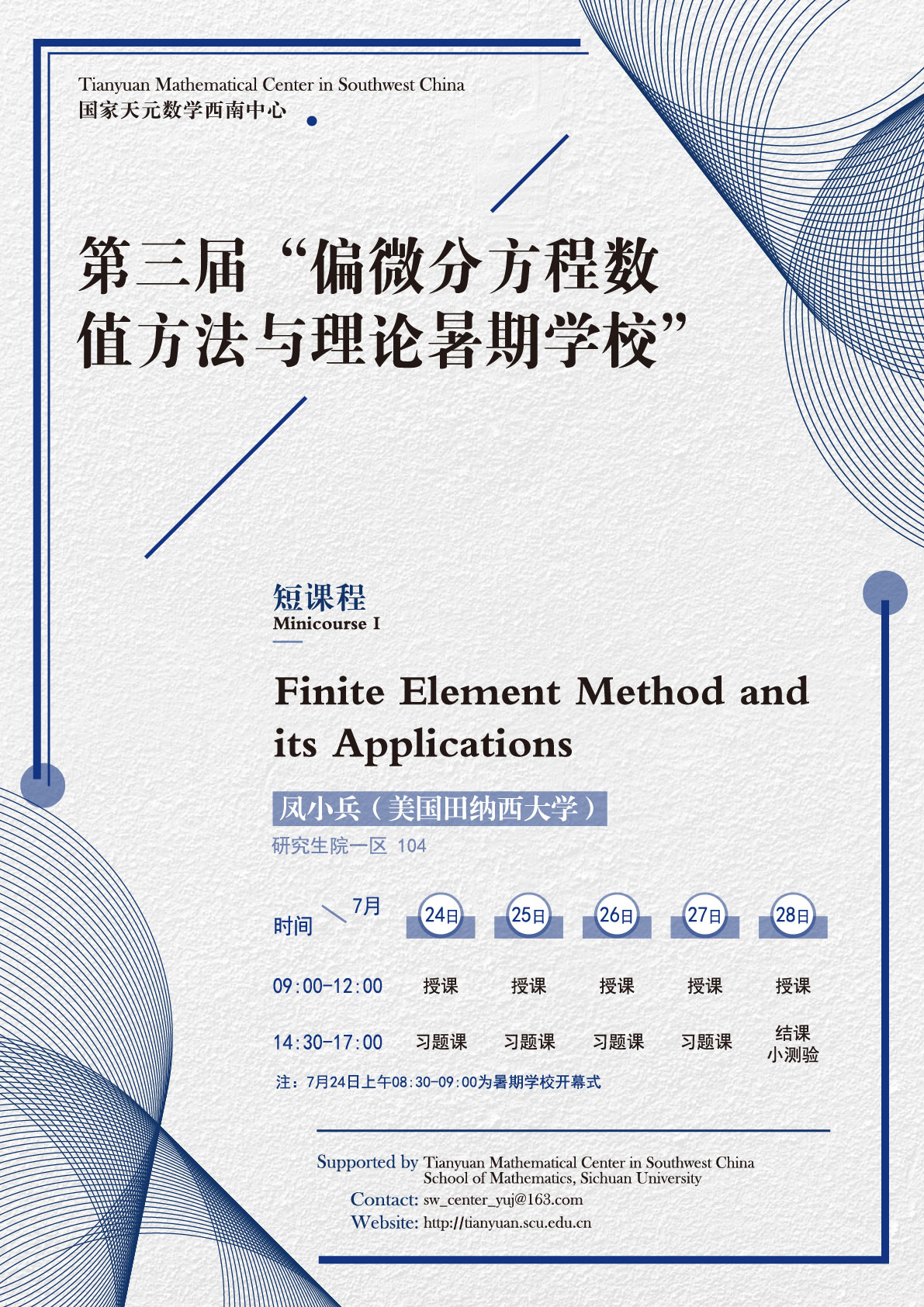Finite Element Method and its Applications

#### 四川大学研究生院一区 104#### abstract

This mini-course intends to present a holistic introduction to the finite element method (FEM), which is arguably the most popular and most powerful numerical method for solving partial differential equations (PDEs). Both boundary value problems (for elliptic PDEs) and initial–boundary value problems (for parabolic and hyperbolic PDEs) will be considered in the course. Following the historic development of the FEM, it will be introduced within a more general Galerkin and Petrov-Galerkin framework. The focuses of the course are to introduce/explain the main ideas/ingredients of the FEM and its variants/extensions (such as nonconforming methods, mixed methods, least squares methods) as well as the main techniques for the convergence analysis. Due to the time constraint, The construction of FE spaces and their interpolation theory will only be briefly covered, so is the Sobolev space theory. Unlike the popular presentation of the materials which assumes the coercivity of the underlying PDE problem (or bilinear form) in a Hilbert space setting, this mini-course adopts an approach which relies on the weak coercivity (i.e., inf-sup/LBB condition) and is formulated in a Banach space setting. If time permits, connection of the FEM to discontinuous Galerkin (DG) methods and mixed finite element methods will also be discussed. Applications of the FEM to be covered include the Stokes and Navier-Stokes equations for incompressible fluids and the Lame’s equations for linear elasticity. Finally, solution methods (i.e., solvers) and computer implementation of the FEM will also be briefly discussed.

#### outline

1) Big picture and sources of PDEs.

2) Formulation and analysis of abstract Galerkin method.

3) Formulation and examples of the finite element method.

4) Convergence theories of the finite element method.

5) Applications to incompressible fluids and linear elasticity.

6*) Solvers and computer implementation.

#### references

1) “Numerical Solutions of Partial Differential Equations by Finite Element Method” by C. Johnson, Dover, 2009.

2) “Theory and Practice of Finite Elements” by A. Ern and J.-L. Guermond, Springer, 2004.

3) ‘The Mathematical Theory of Finite Element Methods” by S. Brenner and R. Scott, Springer, 2008.

4) “The Finite Element Method for Elliptic Problems” by P. G. Ciarlet, SIAM, 2002.

5) “Finite Elements: Theory, Fast Solvers, and Applications in Solid Mechanics” by D. Braess, Cambridge University Press, 2007.

6) “Selected Topics in Finite Element Methods” by Z. Chen and H. Wu, Science Press, 2010.

7) “Survey Lectures on the Mathematical Foundation of the Finite Element Method” by I. Babushka and A. K. Aziz; In: A. K. Aziz, Ed., The Mathematical Foundations of the Finite Element Method with Applications to Partial Differential Equations, Academic Press, New York, 1972, pp. 5-359.

8) “From finite differences to finite elements: A short history of numerical analysis of partial differential equations” by V. Thom\’ee , Journal of Computational and Applied Mathematics, 128:1-54, 2001.

9) “Programming of Finite Element Methods in MATLAB” by L. Chen, https://arxiv.org/abs/1804.05156/

10) “Discontinuous Galerkin finite element differential calculus and applications to numerical solutions of linear and nonlinear PDEs” by X. Feng, T. Lewis and M. Neilan, J. Computational and Applied Mathematics 299:68–91, 2016. A Matlab Toolbox was developed by Stefan Schnake which can be downloaded from

http://www2.math.ou.edu/~sschnake/research

#### VIDEOS

• Finite Element Method and its Applications I
• 09:00 - 10:30, 2019-07-24 at 四川大学研究生院一区 104
• 凤小兵（美国田纳西大学）
• Finite Element Method and its Applications II
• 10:40 - 12:00, 2019-07-24 at 四川大学研究生院一区 104
• 凤小兵（美国田纳西大学）
• Finite Element Method and its Applications III
• 09:00 - 10:30, 2019-07-25 at 四川大学研究生院一区 104
• 凤小兵（美国田纳西大学）
• Finite Element Method and its Applications IV
• 10:40 - 12:00, 2019-07-25 at 四川大学研究生院一区 104
• 凤小兵（美国田纳西大学）
• Finite Element Method and its Applications V
• 09:00 - 10:30, 2019-07-26 at 四川大学研究生院一区 104
• 凤小兵（美国田纳西大学）
• Finite Element Method and its Applications VI
• 10:40 - 12:00, 2019-07-26 at 四川大学研究生院一区 104
• 凤小兵（美国田纳西大学）
• Finite Element Method and its Applications VII
• 09:00 - 10:30, 2019-07-27 at 四川大学研究生院一区 104
• 凤小兵（美国田纳西大学）
• Finite Element Method and its Applications VIII
• 10:40 - 12:00, 2019-07-27 at 四川大学研究生院一区 104
• 凤小兵（美国田纳西大学）
• Finite Element Method and its Applications IX
• 09:00 - 10:30, 2019-07-28 at 四川大学研究生院一区 104
• 凤小兵（美国田纳西大学）
• Finite Element Method and its Applications X
• 10:40 - 12:00, 2019-07-28 at 四川大学研究生院一区 104
• 凤小兵（美国田纳西大学）